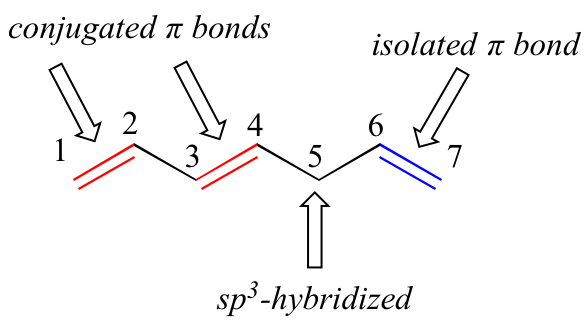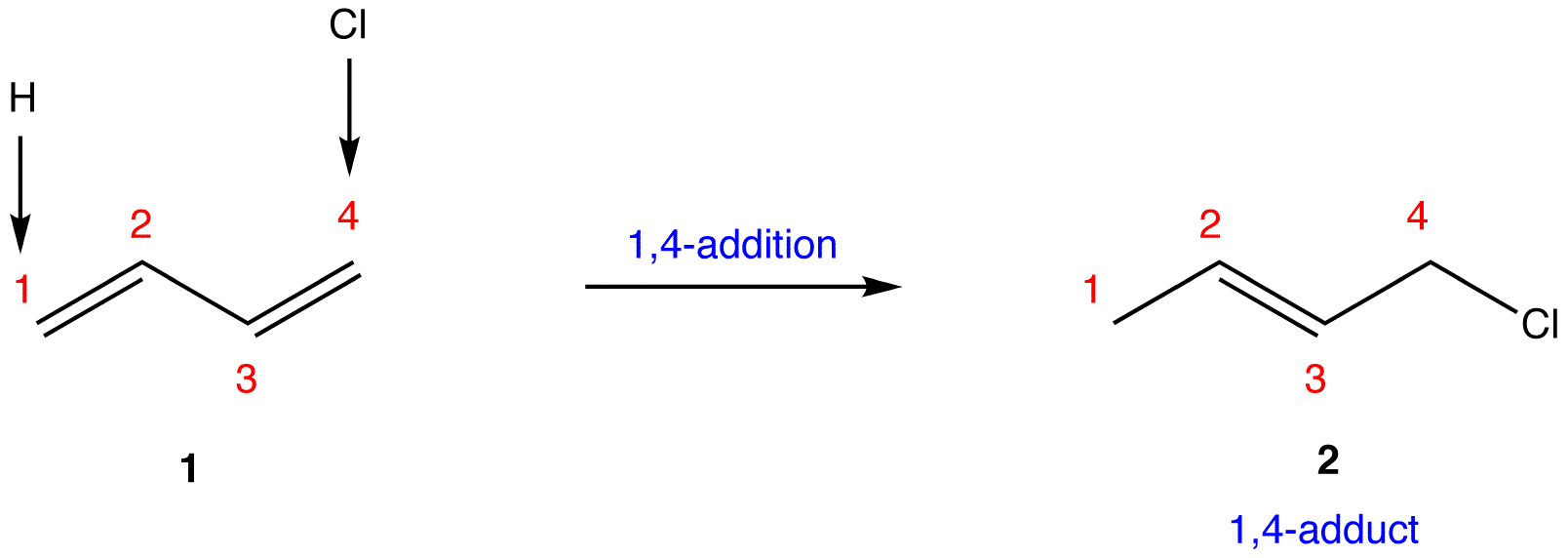# 13.4: Conjugated π-systems

$$\newcommand{\vecs}{\overset { \rightharpoonup} {\mathbf{#1}} }$$ $$\newcommand{\vecd}{\overset{-\!-\!\rightharpoonup}{\vphantom{a}\smash {#1}}}$$$$\newcommand{\id}{\mathrm{id}}$$ $$\newcommand{\Span}{\mathrm{span}}$$ $$\newcommand{\kernel}{\mathrm{null}\,}$$ $$\newcommand{\range}{\mathrm{range}\,}$$ $$\newcommand{\RealPart}{\mathrm{Re}}$$ $$\newcommand{\ImaginaryPart}{\mathrm{Im}}$$ $$\newcommand{\Argument}{\mathrm{Arg}}$$ $$\newcommand{\norm}{\| #1 \|}$$ $$\newcommand{\inner}{\langle #1, #2 \rangle}$$ $$\newcommand{\Span}{\mathrm{span}}$$ $$\newcommand{\id}{\mathrm{id}}$$ $$\newcommand{\Span}{\mathrm{span}}$$ $$\newcommand{\kernel}{\mathrm{null}\,}$$ $$\newcommand{\range}{\mathrm{range}\,}$$ $$\newcommand{\RealPart}{\mathrm{Re}}$$ $$\newcommand{\ImaginaryPart}{\mathrm{Im}}$$ $$\newcommand{\Argument}{\mathrm{Arg}}$$ $$\newcommand{\norm}{\| #1 \|}$$ $$\newcommand{\inner}{\langle #1, #2 \rangle}$$ $$\newcommand{\Span}{\mathrm{span}}$$

### Learning Objectives

After completing this section, you should be able to

1. write a reaction sequence to show a convenient method for preparing a given conjugated diene from an alkene, allyl halide, alkyl dihalide or alcohol (diol).
2. compare the stabilities of conjugated and nonconjugated dienes, using evidence obtained from hydrogenation experiments.
3. discuss the bonding in a conjugated diene, such as 1,3-butadiene, in terms of the hybridization of the carbon atoms involved.
4. discuss the bonding in 1,3-butadiene in terms of the molecular orbital theory, and draw a molecular orbital for this and similar compounds.

### Key Terms

Make certain that you can define, and use in context, the key terms below.

• delocalized electrons
• nodeNext, we’ll consider the 1,3-butadiene molecule. From valence orbital theory alone we might expect that the C2-C3 bond in this molecule, because it is a sigma bond, would be able to rotate freely.Experimentally, however, it is observed that there is a significant barrier to rotation about the C2-C3 bond, and that the entire molecule is planar. In addition, the C2-C3 bond is 148 pm long, shorter than a typical carbon-carbon single bond (about 154 pm), though longer than a typical double bond (about 134 pm).

Molecular orbital theory accounts for these observations with the concept of delocalized pi bonds. In this picture, the four 2p atomic orbitals combine mathematically to form four pi molecular orbitals of increasing energy. Two of these – the bonding pi orbitals – are lower in energy than the p atomic orbitals from which they are formed, while two – the antibonding pi* orbitals – are higher in energy.The lowest energy molecular orbital, pi1, has only constructive interaction and zero nodes. Higher in energy, but still lower than the isolated p orbitals, the pi2 orbital has one node but two constructive interactions – thus it is still a bonding orbital overall. Looking at the two antibonding orbitals, pi3* has two nodes and one constructive interaction, while pi4* has three nodes and zero constructive interactions.

By the aufbau principle, the four electrons from the isolated 2pz atomic orbitals are placed in the bonding pi1 and pi2 MO’s. Because pi1 includes constructive interaction between C2 and C3, there is a degree, in the 1,3-butadiene molecule, of pi-bonding interaction between these two carbons, which accounts for its shorter length and the barrier to rotation. The valence bond picture of 1,3-butadiene shows the two pi bonds as being isolated from one another, with each pair of pi electrons ‘stuck’ in its own pi bond. However, molecular orbital theory predicts (accurately) that the four pi electrons are to some extent delocalized, or ‘spread out’, over the whole pi system.1,3-butadiene is the simplest example of a system of conjugated pi bonds. To be considered conjugated, two or more pi bonds must be separated by only one single bond – in other words, there cannot be an intervening sp3-hybridized carbon, because this would break up the overlapping system of parallel p orbitals. In the compound below, for example, the C1-C2 and C3-C4 double bonds are conjugated, while the C6-C7 double bond is isolated from the other two pi bonds by sp3-hybridized C5.A very important concept to keep in mind is that there is an inherent thermodynamic stability associated with conjugation. This stability can be measured experimentally by comparing the heat of hydrogenation of two different dienes. (Hydrogenation is a reaction type that we will learn much more about in chapter 15: essentially, it is the process of adding a hydrogen molecule – two protons and two electrons – to a p bond). When the two conjugated double bonds of 1,3-pentadiene are ‘hydrogenated’ to produce pentane, about 225 kJ is released per mole of pentane formed. Compare that to the approximately 250 kJ/mol released when the two isolated double bonds in 1,4-pentadiene are hydrogenated, also forming pentane.

The formation of synthetic polymers from dienes such as 1,3-butadiene and isoprene is discussed in Section 18.5. Synthetic polymers are large molecules made up of smaller repeating units. You are probably somewhat familiar with a number of these polymers; for example, polyethylene, polypropylene, polystyrene and poly(vinyl chloride).Figure 13.6: Energy diagram for the hydrogenation of 1,3-butadiene (not to scale).

As the hydrogenation of 1,3-butadiene releases less than the predicted amount of energy, the energy content of 1,3-butadiene must be lower than we might have expected. In other words, 1,3-butadiene is more stable than its formula suggests.

The conjugated diene is lower in energy: in other words, it is more stable. In general, conjugated pi bonds are more stable than isolated pi bonds.

Here is an energy diagram comparing different types of bonds with their heats of hydrogenation (per mole) to show relative stability of each molecule (1 kcal = 4.18 kJ).  (The lower the heat of hydrogenation (per pi bond), the more stable the structure is.)The stabilization of dienes by conjugation is less dramatic than the aromatic stabilization of benzene. Nevertheless, similar resonance and molecular orbital descriptions of conjugation may be written.

### Synthesis of dienes

The two most frequent ways to synthesize conjugated dienes are dehydration of alcohols and dehydrohalogenation of organohalides, which were introduced in the preparation of alkenes (Section 9.9). The following scheme illustrates some of the routes to preparing a conjugated diene.Conjugated pi systems can involve oxygen and nitrogen atoms as well as carbon. In the metabolism of fat molecules, some of the key reactions involve alkenes that are conjugated to carbonyl groups.MO theory is very useful in explaining why organic molecules that contain extended systems of conjugated pi bonds often have distinctive colors. beta-Carotene, the compound responsible for the orange color of carrots, has an extended system of 11 conjugated pi bonds.### Exercises

Exercise 2.9: Identify all conjugated and isolated double bonds in the structures below. For each conjugated pi system, specify the number of overlapping p orbitals, and how many pi electrons are shared among them.Exercise 2.10: Identify all isolated and conjugated pi bonds in lycopene, the red-colored compound in tomatoes. How many pi electrons are contained in the conjugated pi system?Solutions to exercises

## Reactions of dienes

eg:  Two electrophilic addition reactions could occur between 1,3-butadiene (1) and hydrogen chloride.In Reaction 1, the net reaction is addition of a hydrogen atom to C-1 and a chlorine atom to C-4 in 1.  Hence, Reaction 1 is called 1,4-addition and its product (2) 1,4-adduct.In Reaction 2, the net reaction is addition of a hydrogen atom to C-1 and a chlorine atom to C-2 in 1. Hence, Reaction 2 is called 1,2-addition and its product (3) 1,2-adduct.The regioselectivity of the overall reaction depends on the temperature.  At low temperature (eg: –78 °C), the major product is 3; at high temperature (Δ), it is 2.  The carbon-carbon double bond in 2 is more highly substituted than the one in 3, so 2 is more stable than 3.  That the less stable 3 is the major product at low temperature implies that at low temperature the system is under kinetic control and 3 is the faster-forming product. That the more stable 2 is the major product at high temperature means the system is under thermodynamic control.

### Mechanism at low temperatureThe first step is reversible; the second step is irreversible. Thus, the overall reaction is irreversible, i.e, the system is under kinetic control and the major product is the faster-forming product. The first step, an acid-base reaction, generates the allylic carbocation 4 and the chloride ion.  In the second step, 4 reacts with the chloride ion.  In the two resonance forms (4a, 4b) of 4, 4a has the more stable carbocation center and, therefore, is more stable than 4b. Thus, in the hybrid the partial positive charge on C-2 is more intense than that on C-4.In the second step, the chloride ion reacts faster with the more electrophilic C-2, leading to 3. Thus, 3 is the faster-forming product and, since the system is under kinetic control, major product.

### Mechanism at high temperatureNotice that, at high temperature, 2 and 3 interconvert via 4.Since 2 is more stable than 3, the equilibrium lies toward 2 and the equilibrium constant, K, is greater than 1.Hence, 2 is the major product.

### 1,4-Addition, also known as conjugate addition, is a nucleophilic addition reaction of α, β–unsaturated carbonyl compounds and α, β–unsaturated nitriles.

eg:  Two nucleophilic addition reaction could occur between methylvinyl ketone (1) and methanethiol in basic medium.mechanism of Reaction 1:The net reaction from 1 to 4 is the addition of two ligands to atoms 1 and 4 in 1. Hence, the reaction is called 1,4-addition, or conjugate addition, and its product (2) 1,4-adduct.mechanism of Reaction 2:The net reaction from 1 to 3 is the addition of two ligands to atoms 1 and 2 in 1.  Hence, the reaction is called 1,2-addition, or direct addition, and the product (3) a 1,2-adduct.The overall reaction between an α, β–unsaturated compound and a nucleophile is regioselective. Whether the dominant process is 1,4-addition or 1,2-addition depends on several factors, such as the alkene-containing reactant (the α, β–unsaturated compound), nucleophile, solvent, concentration, temperature, reaction time, and catalyst, if any, making if difficult to make a generalization. Most resonance-stabilized carbon nucleophiles, such as enolate ions and enamines overwhelmingly prefer 1,4-addition to 1,2-addition. (See Michael addition)A YouTube element has been excluded from this version of the text. You can view it online here: http://pb.libretexts.org/ochem2walker/?p=58